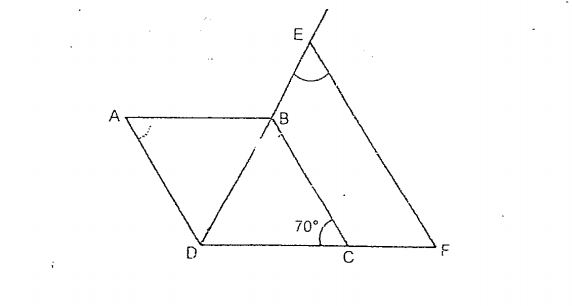# Question 1 of 26

The figure below is not drawn to scale. ABCD is a rhombus. DBE is a straight line. BC is parallel to EF. Find $\angle$DEF.A
53$^\circ$
B
55$^\circ$
C
57$^\circ$
D
59$^\circ$
E
None of the above